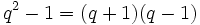# General linear group of degree two

## Definition

The general linear group of degree two over a field$k$ (respectively, over a unital ring$R$), is defined as the group, under multiplication, of invertible$2 \times 2$ matrices with entries in$k$. It is denoted$GL(2,k)$ (respectively,$GL(2,R)$).

For a prime power$q$,$GL(2,q)$ or$GL_2(q)$ denotes the general linear group of degree two over the field (unique up to isomorphism) with$q$ elements.

## Particular cases

### Finite fields

Size of field Common name for general linear group of degree two$2$ symmetric group:S3$3$ general linear group:GL(2,3)$4$ general linear group:GL(2,4)$5$ general linear group:GL(2,5)

### Infinite rings and fields

Name of ring/field Common name for general linear group of degree two
Ring of integers$\mathbb{Z}$ general linear group:GL(2,Z)
Field of rational numbers$\mathbb{Q}$ general linear group:GL(2,Q)
Field of real numbers$\mathbb{R}$ general linear group:GL(2,R)
Field of complex numbers$\mathbb{C}$ general linear group:GL(2,C)

## Arithmetic functions

Here,$q$ denotes the order of the finite field and the group we work with is$GL(2,q)$.$p$ is the characteristic of the field, i.e., it is the prime whose power$q$ is.

Function Value Explanation
order$\! q^4 - q^3 - q^2 + q = q(q + 1)(q-1)^2$$q^2 - 1$ options for first row,$q^2 - q$ options for second row.
exponent$\! p(q^2 - 1) = p(q-1)(q+1)$ There is an element of order$q^2 - 1$ and an element of order$p(q - 1)$. All elements have order dividing$p(q - 1)$ or$q^2 - 1$.
number of conjugacy classes$\! q^2 - 1 = (q + 1)(q-1)$ There are$q(q-1)$ conjugacy classes of semisimple matrices and$q - 1$ conjugacy classes of matrices with repeated eigenvalues.

## Group properties

Property Satisfied Explanation
Abelian group No The matrices$\begin{pmatrix} 1 & 1 \\ 0 & 1 \\\end{pmatrix}$ and$\begin{pmatrix} 0 & 1 \\ 1 & 0 \\\end{pmatrix}$ don't commute.
Nilpotent group No$PSL(2,q)$ is simple for$q \ge 4$, and we can check the cases$q = 2, 3$ separately.
Solvable group Yes if$q = 2,3$, no otherwise.$PSL(2,q)$ is simple for$q \ge 4$.
Supersolvable group Yes if$q - 2$, no otherwise.$PSL(2,q)$ is simple for$q \ge 4$, and we can check the cases$q = 2, 3$ separately.

## Subgroup-defining functions

Subgroup-defining function Value Explanation
Center The subgroup of scalar matrices. Cyclic of order$q - 1$ Center of general linear group is group of scalar matrices over center.
Commutator subgroup Except the case of$GL(2,2)$, it is the special linear group of degree two, which has index$q - 1$. Commutator subgroup of general linear group is special linear group

## Quotient-defining functions

Subgroup-defining function Value Explanation
Inner automorphism group Projective general linear group of degree two Quotient by the center, which is the group of scalar matrices.
Abelianization This is isomorphic to the multiplicative group of the field. Quotient by the commutator subgroup, which is the special linear group, which is the kernel of the determinant map that surjects to the multiplicative group of the field.